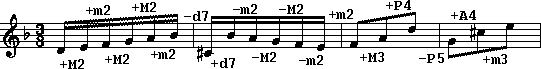# Help for the interval search field in Themefinder

Guidelines for searching by interval:

Intervals consist of three components which must occur in the following order for each interval:

1. interval direction
• The plus sign (+) indicates a rising interval.
• The minus sign (-) indicates a falling interval.
2. interval quality
 d or D = diminished m = minor M = Major A or a = Augmented P or p = Perfect
3. diatonic interval size
 1 = unison 2 = second 3 = third 4 = fourth 5 = fifth 6 = sixth 7 = seventh 8 = octave 9 = ninth = octave + second 10 = tenth = octave + third 11 = eleventh = octave + fourth etc.

Examples:Additional guidelines for searching by interval:
• If a component is missing from the interval, then any of the possible combinations will be searched for. Examples:  m2 will expand to either +m2 or -m2 because the direction of the interval was not specified. -3 will expand to -m3, -M3, -A3, or -d3 because the quality of the interval was not specified. +P will expand to +P4, +P5, +P8, etc. because the size of the interval was not specified. + will match any interval which is rising. M will match any major interval which is either rising or falling. 4 will match any interval with a diatonic size of 4 which is either rising or falling.
• Intervals may be separated by spaces. Separation is optional but is recommended for unusual interval searches. Examples:  +P4-P5+m3-M2 is equivalent to +P4 -P5 +m3 -M2 +P4-P5+m-3-M2 is equivalent to +P4 -P5 +m -3 -M2 +4P-5P+3m-2M is equivalent to +4 P -5 P +3 m -2 M

.
To search for an unknown or arbitrary interval, use the dot to represent any one interval. Example:
 (+M2 . -m3) will match to (+M2 -M2 -m3) (+M2 +P4 -m3) (+M2 -P5 -m3) (+M2  P1 -m3) etc.
?
To indicate an optional interval, append a question mark. Example:
 (+M3 +m3? -m3) will match to (+M3 +m3 -m3) (+M3     -m3) etc.
*
To indicate any number of optional intervals, insert an asterisk. Example:
 (+M2 * -m3) will match to (+M2 -M2 P1 +M6 -m3) (+M2 +P5 -m3) (+M2 -P4 +m6 -m2 -m2 -m3) (+M2 +m7 -P8 +M2 -m3) etc.
^
A caret appended to an interval indicates an optional inversion of the interval. The inverted interval is in the opposite direction. Examples:
 (+M3 +P4^ -m2) will match to (+M3 +P4 -m2) (+M3 -P5 -m2) (+P4 +M3^ -P5) will match to (+P4 +M3 -P5) (+P4 -m6 -P5) (+m2 A4^ +M2) will match to (+m2 +A4 +M2) (+m2 -d5 +M2) (+m2 -A4 +M2) (+m2 +d5 +M2) (+m6 -3^ -M2) will match to (+m6 -M3 -M2) (+m6 +m6 -M2) (+m6 -m3 -M2) (+m6 +M6 -M2) (+m6 -d3 -M2) (+m6 +A6 -M2) (+m6 -A3 -M2) (+m6 +d6 -M2)
#
To search for an interval or its inversion in the same direction, append the number symbol. Examples:
 (+M3 +P4# -m2) will match to (+M3 +P4 -m2) (+M3 +P5 -m2) (+P4 +M3^ -P5) will match to (+P4 +M3 -P5) (+P4 +m6 -P5) (+m2 A4^ +M2) will match to (+m2 +A4 +M2) (+m2 +d5 +M2) (+m2 -A4 +M2) (+m2 -d5 +M2) (+m6 -3^ -M2) will match to (+m6 -M3 -M2) (+m6 -m6 -M2) (+m6 -m3 -M2) (+m6 -M6 -M2) (+m6 -d3 -M2) (+m6 -A6 -M2) (+m6 -A3 -M2) (+m6 -d6 -M2)
```

```